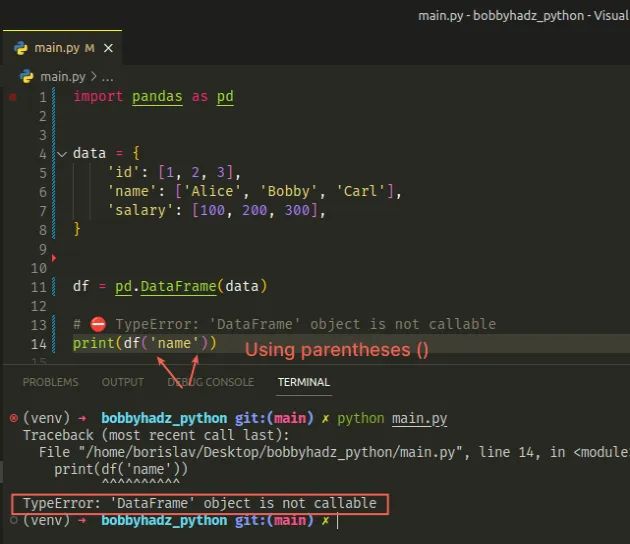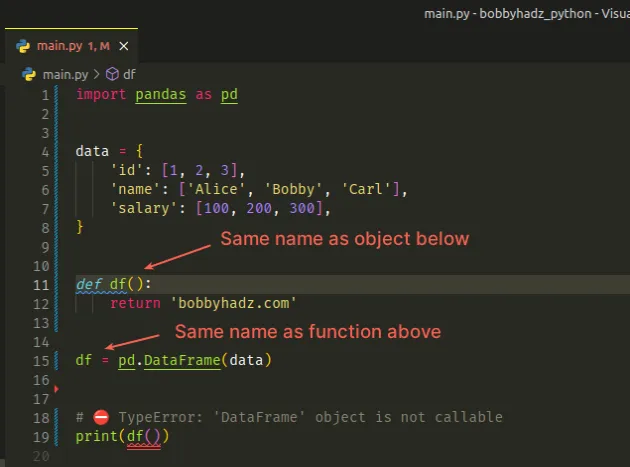# TypeError: 'DataFrame' object is not callable error [Solved]Last updated: Feb 25, 2023
4 min## #TypeError: 'DataFrame' object is not callable error [Solved]

The Python "TypeError: 'DataFrame' object is not callable" error occurs when we use parentheses `()` instead of square brackets `[]` to access a column in a `DataFrame`.

To solve the error, make sure to use square brackets when accessing the column.

Assume you have the following DataFrame object.

main.py
```Copied!```import pandas as pd

data = {
'id': [1, 2, 3],
'name': ['Alice', 'Bobby', 'Carl'],
'salary': [100, 200, 300],
}

df = pd.DataFrame(data)

print(df)
``````

The code sample produces the following output.

shell
```Copied!```   id   name  salary
0   1  Alice     100
1   2  Bobby     200
2   3   Carl     300
``````

If you try to access a specific column using parentheses `()`, you'd get an error.

main.py
```Copied!```import pandas as pd

data = {
'id': [1, 2, 3],
'name': ['Alice', 'Bobby', 'Carl'],
'salary': [100, 200, 300],
}

df = pd.DataFrame(data)

# ⛔️ TypeError: 'DataFrame' object is not callable
df('name') # 👈️ using parentheses ()
``````We used parentheses `()` instead of square brackets `[]` when accessing the column which caused the error.

## #Use square when getting a subset of a DataFrame's rows and columns

Instead, use square brackets `[]` to select a subset of a `DataFrame`.

main.py
```Copied!```import pandas as pd

data = {
'id': [1, 2, 3],
'name': ['Alice', 'Bobby', 'Carl'],
'salary': [100, 200, 300],
}

df = pd.DataFrame(data)

print(df['name']) # ✅ using square brackets []
``````

Notice that we used square brackets `[]` when accessing the `name` column.

The code sample produces the following output.

shell
```Copied!```0    Alice
1    Bobby
2     Carl
Name: name, dtype: object
``````

## #Calling a method with the values in a column

If you need to call a method with the values in a column, use square brackets `[]` when accessing the column and parentheses `()` to invoke the method.

main.py
```Copied!```import pandas as pd

data = {
'id': [1, 2, 3],
'name': ['Alice', 'Bobby', 'Carl'],
'salary': [100, 200, 300],
}

df = pd.DataFrame(data)

print(df['salary'].mean())  # 👉️ 200.0
``````

We used square brackets `[]` to access the `salary` column and then called the `mean()` method using parentheses.

Keys in a dictionary or columns in a `DataFrame` are accessed using square brackets `[]`.

Functions and methods are invoked using parentheses `()`.

## #Slicing a DataFrame object

If you need to get a subset of a `DataFrame` object, use square brackets.

main.py
```Copied!```import pandas as pd

data = {
'id': [1, 2, 3],
'name': ['Alice', 'Bobby', 'Carl'],
'salary': [100, 200, 300],
}

df = pd.DataFrame(data)

print(df[0:2])
``````

The code sample returns the first 2 rows of the `DataFrame` object.

output
```Copied!```   id   name  salary
0   1  Alice     100
1   2  Bobby     200
``````

## #Accessing a specific column after slicing

You can also access a specific column after the slicing operation.

main.py
```Copied!```import pandas as pd

data = {
'id': [1, 2, 3],
'name': ['Alice', 'Bobby', 'Carl'],
'salary': [100, 200, 300],
}

df = pd.DataFrame(data)

print(df[0:2]['name'])
``````

The code sample produces the following output.

output
```Copied!```0    Alice
1    Bobby
Name: name, dtype: object
``````

We only selected the first and second rows of the `name` column in the `DataFrame`.

## #Calling a method after selecting a subset of rows

If you need to call a method with a subset of the rows of a column, use parentheses.

main.py
```Copied!```import pandas as pd

data = {
'id': [1, 2, 3],
'name': ['Alice', 'Bobby', 'Carl'],
'salary': [100, 200, 300],
}

df = pd.DataFrame(data)

print(df[0:2]['salary'].mean())  # 👉️ 150.0
``````

We selected the first 2 rows of the `salary` column and then called the `mean()` method on the values.

## #Calculating the variance over an axis

If you need to calculate the variance over an axis, use the pandas.DataFrame.var method.

main.py
```Copied!```import pandas as pd

data = {
'id': [1, 2, 3],
'name': ['Alice', 'Bobby', 'Carl'],
'height': [1.61, 1.87, 1.49]
}

df = pd.DataFrame(data)

print(df.var())
``````

The code sample produces the following output.

shell
```Copied!```id        1.000000
height    0.037733
dtype: float64
``````

By default, the `ddof` (Delta Degrees of Freedom) argument is set to `N - ddof` where N is the number of elements.

You can set `ddof` to `0` to normalize by `N` instead of `N - 1`.

main.py
```Copied!```import pandas as pd

data = {
'id': [1, 2, 3],
'name': ['Alice', 'Bobby', 'Carl'],
'height': [1.61, 1.87, 1.49]
}

df = pd.DataFrame(data)

# id        0.666667
# height    0.025156
# dtype: float64
print(df.var(ddof=0))
``````

The code sample produces the following output.

output
```Copied!```id        0.666667
height    0.025156
dtype: float64
``````

## #Clashing names of a function and a `DataFrame` object

Another common cause of the error is having a function with the same name as the variable that stores your `DataFrame` object.

main.py
```Copied!```import pandas as pd

data = {
'id': [1, 2, 3],
'name': ['Alice', 'Bobby', 'Carl'],
'salary': [100, 200, 300],
}

def df():

df = pd.DataFrame(data)

# ⛔️ TypeError: 'DataFrame' object is not callable
print(df())
``````We initially named a function `df` and then declared a `df` variable, setting it to a `DataFrame` object.

When we try to call the `df` variable, we actually end up trying to call the `DataFrame` object which causes the error.

To solve the error, rename the function or the `DataFrame` object so that the names don't clash.

main.py
```Copied!```import pandas as pd

data = {
'id': [1, 2, 3],
'name': ['Alice', 'Bobby', 'Carl'],
'salary': [100, 200, 300],
}

def df():

# 👇️ renamed variable
my_df = pd.DataFrame(data)

``````

I renamed the variable, so it doesn't clash with the function anymore.

The `df()` line now calls the function as expected.